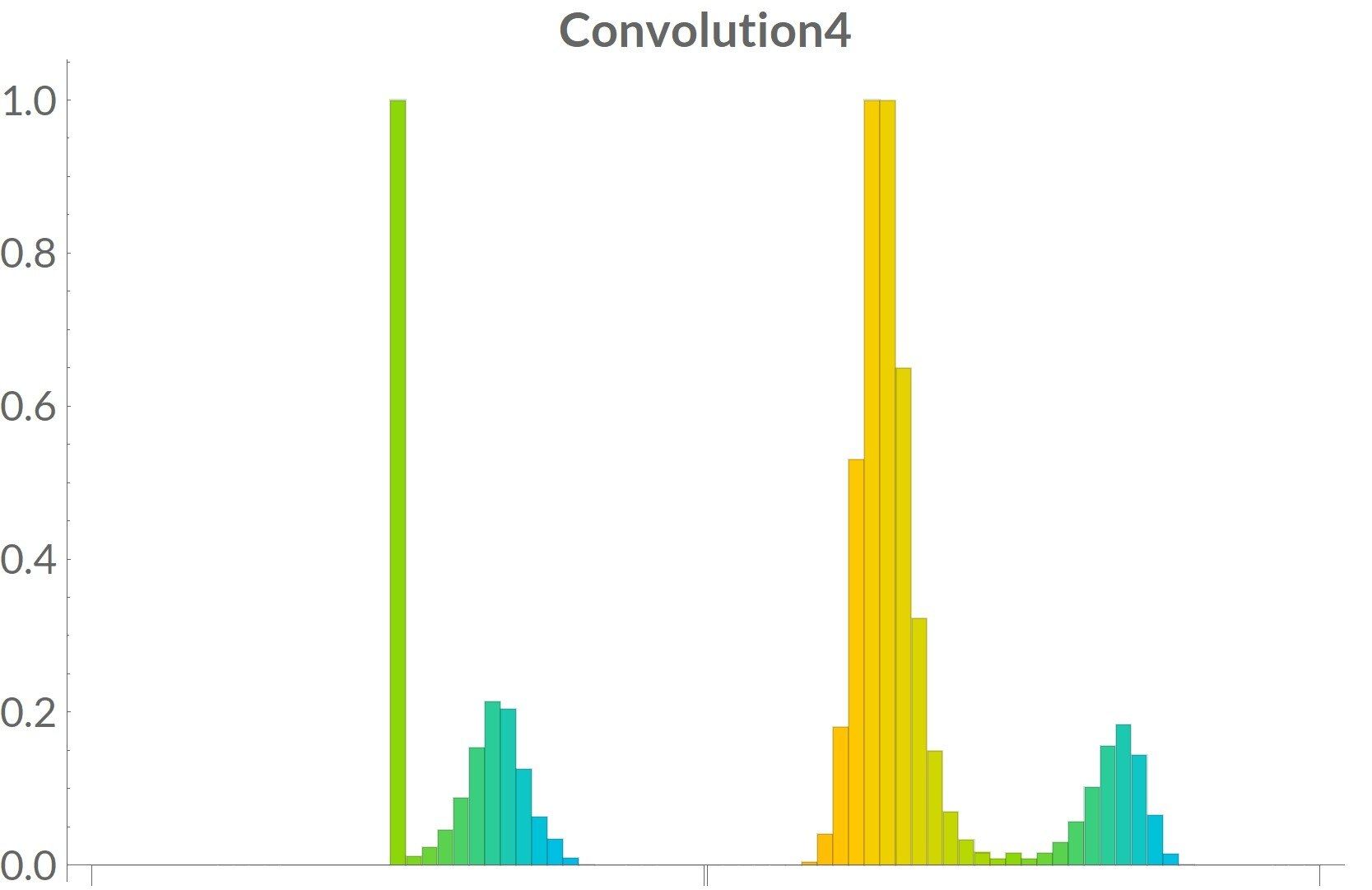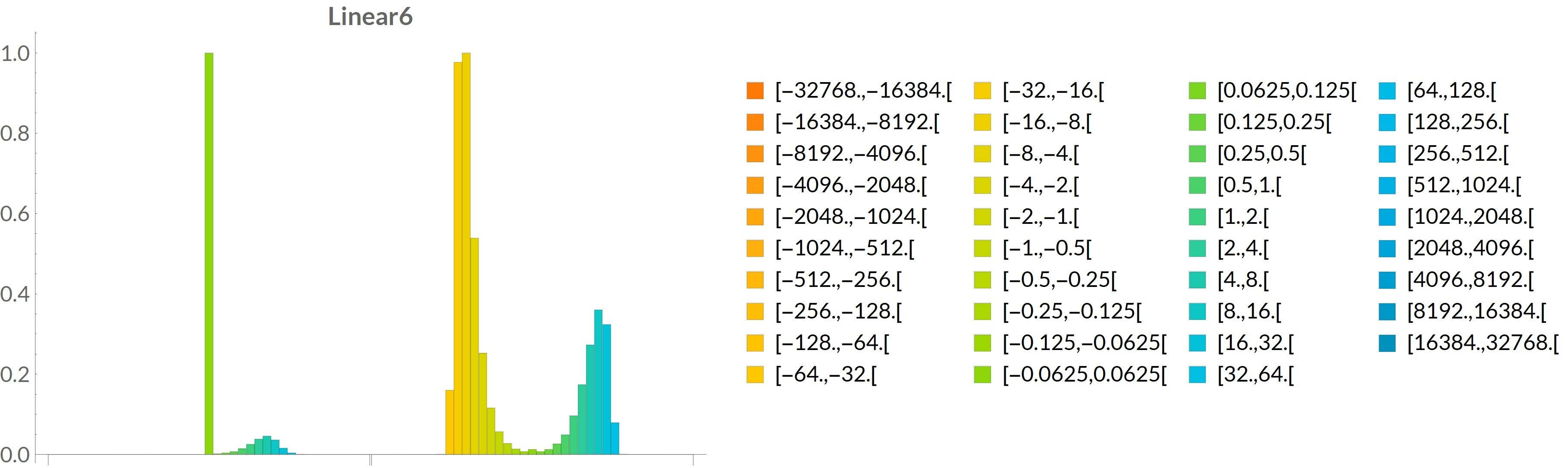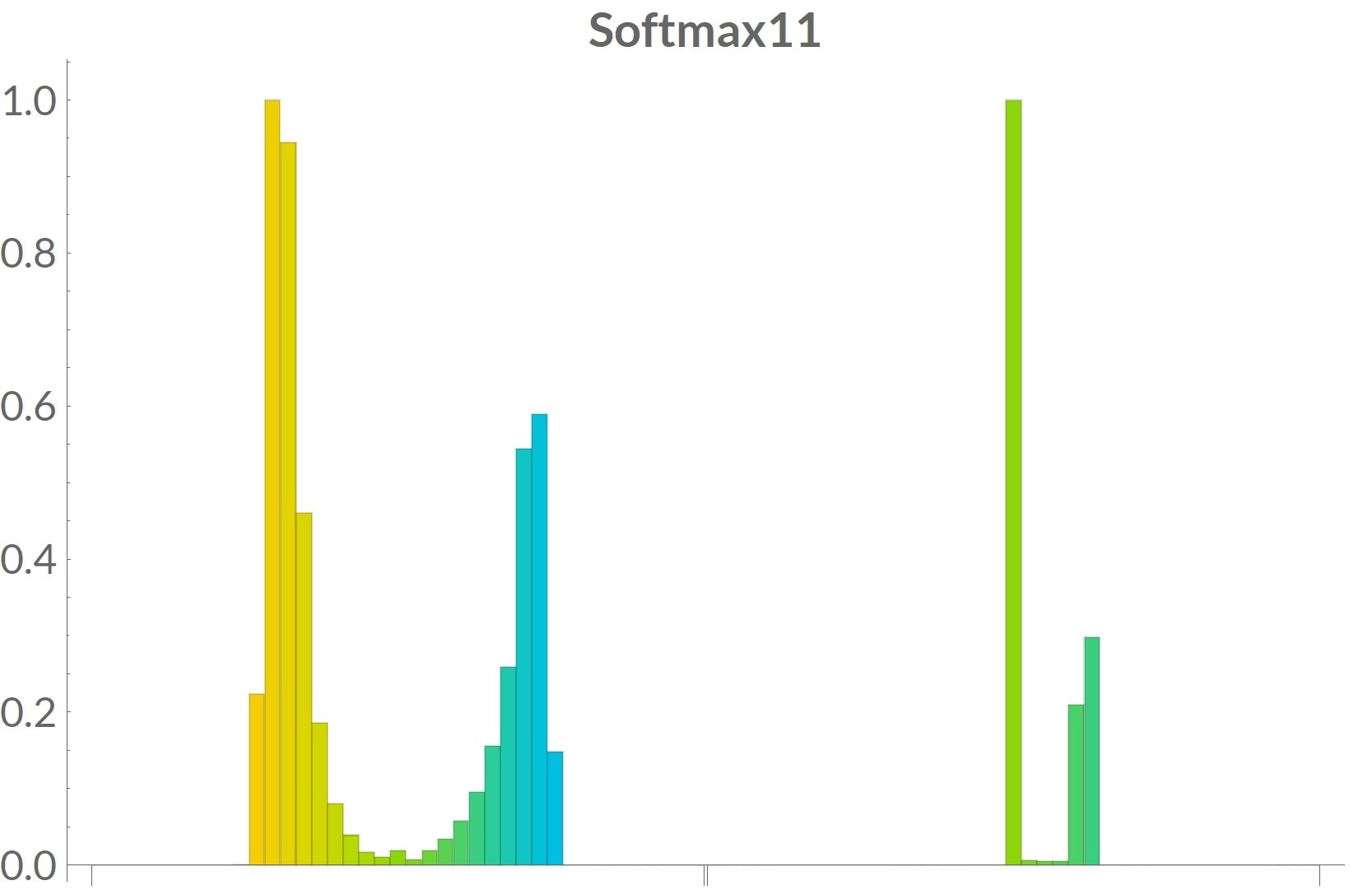## Compute activation statistics

To quantize the input/output of the network layers, you need to know the range of values. This means that you will need to evaluate the network on several patterns and record the values at the input and output of each layer. Ideally, the full set of training patterns should be used.

You probably do not want to keep all the values, because it is a lot of data if you evaluate the network on all the training patterns.

In that case, the simplest solution would be to keep the min and the max values. However, you may want to have more information about the distribution of values to experiment with several quantization scheme later.

So another possibility is to compute a histogram for each input and output, and upgrade it each time the network is evaluated on a new training pattern. Because you are only interested in the number of bits that are required to represent the values, the bins of the histogram can be based upon powers of two.

In the images below, you can see some histograms of the activations of some layers of the KWS network. In each image, the left shows the input of a layer and the output is on the right. The scale is logarithmic.Convolution4 is showing the input of a convolution layer on the left part of the picture. Since this input is also the output of a previous ReLU layer then it is highly asymmetric which is visible on the graph.The horizontal scale is the same for all pictures. As we can see on this picture, power of two values were used as the bounds of the different histogram bins.